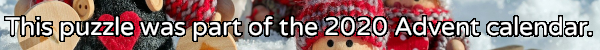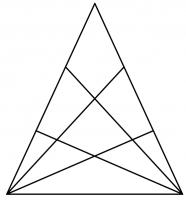mscroggs.co.uk
mscroggs.co.uksubscribe

# Puzzles

## 25 DecemberIt's nearly Christmas and something terrible has happened: you've just landed in a town in the Arctic circle with a massive bag of letters for Santa, but you've lost to instructions for how to get to Santa's house near the north pole. You need to work out where he lives and deliver the letters to him before Christmas is ruined for everyone.
Due to magnetic compasses being hard to use near the north pole, you brought with you a special Advent compass. This compass has nine numbered directions. Santa has given the residents of the town clues about a sequence of directions that will lead to his house; but in order to keep his location secret from present thieves, he gave each resident two clues: one clue is true, and one clue is false.
Here are the clues:
9
"The 4th digit is 4."
"The 8th digit is 4."
4
"The 3rd digit is 1."
"The 4th digit is 7."
10
"The 11th digit is a factor of 888."
"The 11th digit is a factor of 88."
13
"The 3rd digit is 1."
"The 4th digit is 6."
23
"The 10th digit is 4."
"The 10th digit is 3."
1
"The last digit is 1, 9 or 5."
"The last digit is 1."
5
"The 3rd digit is 1."
"The 4th digit is 3."
16
"The 5th digit is 3."
"The 6th digit is 3."
11
"The sequence's length is 2+1+6."
"The sequence's length is 2×1×6."
12
"The 6th to 8th digits are 3, 3, 4."
"The 7th to 9th digits are 3, 3, 4."
2
"The 7th digit is 4."
"The 8th digit is 4."
21
"The 5th digit is 3."
"The 9th digit is 9."
22
"The 9th digit is 9."
"The 10th digit is 4."
17
"The 3rd digit is 1."
"The 4th digit is 9."
15
"The 2nd* digit is 7."
"The 1st digit is 8."
14
"The 1st digit is 7."
"The 2nd digit is 7."
7
"The last digit is not 5."
"The 4th digit is 5."
3
"The 11th digit is a factor of 10."
"The 11th digit is a factor of 321."
6
"The sequence's length is 1+9+2."
"The sequence's length is 1×9×2."
20
"The 5th digit is 3."
"The 5th digit is 4."
19
"5 does not appear."
"5 appears exactly once."
24
"The 3rd digit is 1."
"The 4th digit is 8."
25
18
"The 1st digit is 3, 7 or 8."
"The sequence's length is prime."
8
"The 3rd digit is 1."
"The 4th digit is 2."
You can view the map here.

## 24 DecemberThere are six ways to put two tokens in a 3 by 3 grid so that the diagonal from the top left to the bottom right is a line of symmetry:
Today's number is the number of ways of placing two tokens in a 29 by 29 grid so that the diagonal from the top left to the bottom right is a line of symmetry.

## 23 December198 is the smallest number that is equal to 11 times the sum of its digits.
Today's number is the smallest number that is equal to 48 times the sum of its digits.

## 22 DecemberPut the digits 1 to 9 (using each digit exactly once) in the boxes so that the sums are correct. The sums should be read left to right and top to bottom ignoring the usual order of operations. For example, 4+3×2 is 14, not 10. Today's number is the largest number you can make with the digits in the red boxes.
 + + = 18 + + + ÷ - = 1/2 + + + + ÷ = 3/2 =24 =8 =13
Tags: numbers, grids

## 21 DecemberThere are 3 ways to order the numbers 1 to 3 so that no number immediately follows the number one less that itself:
• 3, 2, 1
• 1, 3, 2
• 2, 1, 3
Today's number is the number of ways to order the numbers 1 to 6 so that no number immediately follows the number one less that itself.

## 20 December18 can be written as the sum of 3 consecutive (strictly) positive integers: 5 + 6 + 7.
18 can also be written as the sum of 4 consecutive (strictly) positive integers: 3 + 4 + 5 + 6.
18 is in fact the smallest number that can be written as the sum of both 3 and 4 consecutive (strictly) positive integers.
Today's number is the smallest number that can be written as the sum of both 12 and 13 consecutive (strictly) positive integers.
Tags: numbers, sums

## 19 DecemberThe diagram to the right shows a triangle. Two of the sides of the triangle have been split into three pieces, with lines drawn from the opposite vertex. In total, the diagram now contains 27 triangles of any size.
Another triangle has two of its sides split into eight pieces, with lines drawn from the opposite vertex. How many triangles (of any size) would this create?

## 18 DecemberThe expansion of $$(x+y+z)^3$$ is
$$x^3 + y^3 + z^3 + 3x^2y + 3x^2z + 3xy^2 + 3y^2z + 3xz^2 + 3yz^2 + 6xyz.$$
This has 10 terms.
Today's number is the number of terms in the expansion of $$(x+y+z)^{26}$$.
Tags: algebra

## Archive

Show me a random puzzle
▼ show ▼